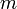# Exercise: Exploring the performance of a cruise controller*

Jump to: navigation, search

In this exercise, you will modify the parameters of a feedback system to explore how changes in parameters affect the performance of the system. To complete this exercise, you will need some basic understanding of how MATLAB and SIMULINK work.

#### Basic version (from print copy)

Download the \matlab{} code used to produce simulations for the cruise control system in Figure 1.13. Using trial and error, change the parameters of the control law so that the overshoot in speed is not more than 1 m/s for a vehicle with mass= 1000 kg.

#### More advanced version

Download the cruise control simulation, which contains a SIMULINK model of a simple speed controller, similar to the one described in Chapter 1 - Introduction. Figure out how to run the example and plot the vehicles speed as a function of time, then complete the following:

1. Leaving the control gains at their default values, plot the response of the system to a step input and measure the time it takes for the system error to settle to within 95% of commanded change in speed (i.e., ±0.5 miles/hour).

2. By manually tuning the control gains, design a controller that settles 50% faster than the default controller. Include the gains you used, a plot of the closed loop response, and describe any undesireable features in the solution you obtain.

3. Change the parameters of the control law so that the overshoot in the speed is not more than 1 m/s for a vehicle with mass= 1000 kg.

All plots should included a title, labeled axes (with units), and reasonable axis limits.

Note: The purpose of this problem is to give you some familiarity with MATLAB and SIMULINK. Don’t worry if you don’t yet know how the control law works or why it does what it does. This will be covered later in the text.